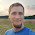## Saturday, January 25, 2014

### Python list, set and dict comprehentions 2.7+

Python supports list comprehension since v2.0. These expressions truly revolutionized python, making various functions much simpler and more readable. Let's see some basic LC in action:

>>> [x for x in "test"]
['t', 'e', 's', 't']

Now often there is a need to generate a set or dict in a similar way, so I often see such code:

>>> set([x for x in "test"])
set(['s', 'e', 't'])
>>> dict([(x,x) for x in "test"])
{'s': 's', 'e': 'e', 't': 't'}

This is good:
- it works!
- it's more readable than implementing a for loop.

But using python 2.7+ You can to it better! The latest python 2.7.X and 3.X support dict and set comprehensions - now this is pythonic! You can achieve the same results the following way:

>>> {x for x in "test"}
set(['s', 'e', 't'])
>>> {x: x for x in "test"}
{'s': 's', 'e': 'e', 't': 't'}

This is excellent!
- it works!
- it's more readable than creating a dict/set from a LC
- it's faster!!!

Simple performance comparison:

>>> timeit.timeit('set([x for x in "test"])')
0.44252514839172363
>>> timeit.timeit('{x for x in "test"}')
0.37139105796813965

>>> timeit.timeit('dict([(x,x) for x in "test"])')
0.8899600505828857
>>> timeit.timeit('{x: x for x in "test"}')
0.3909759521484375

Cheers!
KR

1.Hi Krzysiek,

I was wondering what about memory usage, so I did a test. This is my test result:

In : %memit dict([(x,x) for x in "test"])
peak memory: 19.25 MiB, increment: 0.00 MiB

In : %memit {x: x for x in "test"}
peak memory: 19.25 MiB, increment: 0.00 MiB

Hmm, same result, but if I change test data to:

In : %memit dict([(x,x) for x in data])
peak memory: 228.05 MiB, increment: 130.12 MiB

In : %memit {x: x for x in data}
peak memory: 145.93 MiB, increment: 93.75 MiB

PS. I used ipython, with (memory_profiler, %load_ext memory_profiler)

1.Thanks for the update, this is also an argument for using these expressions.

2.I forgot to specify variable data:

data = range(0, 999999)

2.This comment has been removed by the author.